Structural Bioinformatics Library Template C++ / Python API for developping structural bioinformatics applications.
User Manual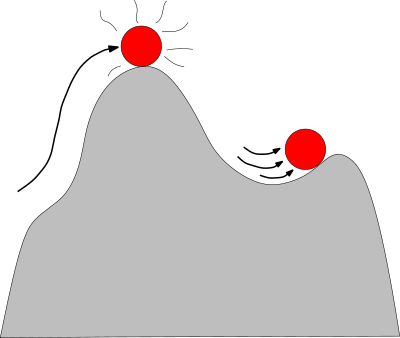# Molecular_potential_energy

Authors: F. Cazals and A. Chevallier and T. Dreyfus

# Introduction

This package defines generic functors to compute the potential energy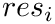of a molecular system:

• Atomic and coarse grain representations are supported.

• The potential energy decomposes as a sum of contributions, which themselves decomposes into terms. The functors give access to contributions and terms.

• Instantiations of these generic functors are provided for several classical force fields, including CHARMM, Amber, Martini. Additional force fields are easily incorporated using the FFXML format, from the OpenMM library.

• Novel force fields are also easily defined by defining terms, which add up to define contributions.

• The ability to support various force fields aims at leveraging three key operations on energy landscapes, namely:

• NB: The computation of potential energy gradients (usually used as forces) using on automatic differentiation tools, will be provided elsewhere.

# Terminology

## Potential energy

To describe the potential energy of a macro-molecular system, we shall use the following terminology:

• particle class (for atoms or pseudo-atoms) : a class of particles. Example for proteins: C-alpha (CA) carbons.

• particle type: a subblass of a particle class, with identical (chemical, physical) properties. The type is in one-to-one correspondence with its so-called identifier. Example: the CA carbon of a given amino-acid.

To describe a force field, we shall need:

• (bonded terms) pairs / triples / quadruples of classes of particle types;

• (non bonded terms) singletons and pairs of particle types.

The following examples stress the roles of particle types versus particle classes.

For an atomic force field as Amber, the class CT of particle types groups the alpha-carbon and the carbons of the side chains with no double valence bond. Each particle type in the class CT has its own identifier–a number, allowing to map the corresponding carbon and its residue to the particle type. While all the carbons of class CT are equivalent for the bonded terms, they may have different set of parameters for the non bonded terms (e.g for their charge).

BLN69 is a protein model involving 69 beads i.e pseudo amino-acids of three types (B, L, N) – see Example Landscapes defined from mathematical functions .

A typical expression foris presented by Eq. (fig-eq-ff), and has three components:

• Bonded contributions: consist of three terms: bond / valence / torsion terms. These terms are best described in terms of internal coordinates.

• Non bonded contribution: typically consists of van der Waals and electrostatic terms.

• Knowledge based terms: terms depending on the presence of specific sub-structures (SSE, native contacts, etc) in a conformation.
Consider a conformation of a macro-molecular system. A potential energy is a real-valued function of the coordinates (internal, cartesian), defined by a sum of contributions , which themselves decompose as a sum of terms . The typical expression is as follows: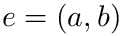with

•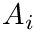: contribution for bonds.

•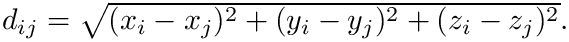: contribution for covalent angles.

•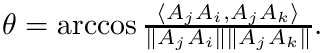: contribution for proper dihedral angles.

•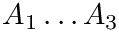: contribution for improper dihedral angles.

•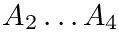: contribution for van der Walls interactions (typically a Lennard-Jones potential).

•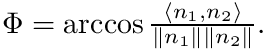: contribution for electrostatic interactions.

• The number of parameters used to compute these six contributions is provided in a 6-tuple denoted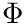.

• We also report the same 6-tuple in which identical values are counted once only, denote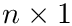. Consider e.g. two carbon types from the same class. If two pairs from this class have the same spring constant, this value is counted once.

The following remarks are in order:

• Covalent terms are expressed with primitive internal coordinates (Molecular_coordinates), while non-covalent terms are expressed with pairwise distances.

• The 6-tuple aims at scaling the complexity of a force field, which depends on the different possible combinations of particle types in a force field. See Examples: instantiating classical force fields .

• Knowledge based information may be incorporated into the aforedefined terms. For example, information on SSE can be incorporated into the contributions for dihedral angles. Below, we illustrate this possibility for the potential energy of the BLN69 protein model.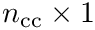## Potential energy: units

The following units are used consistently:

• charge: proton charge
• temperature: Kelvin
• energy: kJ/mol
• force: kJ/mol/nm
• mass: atomic mass units, i.e. daltons
• time: picoseconds

Mechanisms to ensure the compile time coherence of expressions involving such units are provided in the package UnitSystemTraits .

(Conversions) Amongst the previous units, two of them are especially subject to different conventions, namely distances (typically used to store Cartesian coordinates) and angles (in radians or degrees). The remaining ones are mostly used for internal calculations within force fields. To allow users to vary conventions in particular for distances and angles, conversion can be set in the force field loader through the methods SBL::IO::T_Potential_energy_parameters::set_metric_scale_factor and SBL::IO::T_Potential_energy_parameters::set_is_degree .

(Reduced units) At times, units known as dimensionless units or reduced units are used. This allows (i) working with numerical values of the order of unity, (ii) simplifying the equations of motions. Reduced units are mentioned wherever appropriate. In particular, the force field for BLN69 uses reduced units.

## Output: granularity

The potential energydescribed in Eq. (fig-eq-ff) is decomposed in several contributions. This package is designed so that each contribution can be computed separately. This package provides also data structures computing a group of contributions, as for example the sum of the bonded contributions, or the sum of the non bonded contributions.

# Generic force field representation

## Bond length terms

There is a unique class provided for computing the harmonic bond length term that is SBL::CSB::T_Molecular_potential_energy_bond_length_term_harmonic . For two bonded particles, the formula is :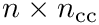where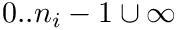is a force constant,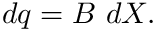is an equilibrium distance constant, and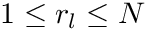is the distance between the particles.

## Valence angle terms

Two classes are provided to compute the harmonic bond angle term :

1. Harmonic valence angle term : SBL::CSB::T_Molecular_potential_energy_bond_angle_term_harmonic . For three bonded particles, the formula is :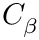withthe force constant,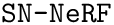the equilibrium angle constant, and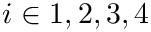the valence angle formed by the three bonded particles.

2. Urey Bradley term : SBL::CSB::T_Molecular_potential_energy_bond_angle_term_Urey_Bradley . For three bonded particles, the formula is :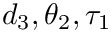withthe force constant,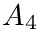the equilibrium distance constant, and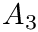the distance between the first and third particles of the valence angle formed by the three bonded particles.

## Torsion angle terms

There are four classes provided for computing the possible torsion angle terms :

1. Harmonic torsion angle term : SBL::CSB::T_Molecular_potential_energy_torsion_angle_term_harmonic . For four bonded particles, the formula is :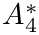whereis a force constant,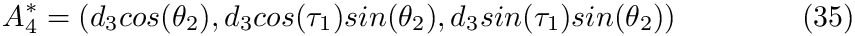is an equilibrium angle constant, and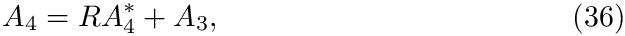is a torsion angle formed by the four bonded particles.

2. Periodic torsion angle term : SBL::CSB::T_Molecular_potential_energy_torsion_angle_term_periodic . For four bonded particles, the formula is :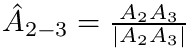where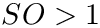is the periodicity of the term,is a force constant,is a phase shift angle, andis a torsion angle formed by the four bonded particles.

3. Fourier torsion angle term : SBL::CSB::T_Molecular_potential_energy_torsion_angle_term_Fourier . For four bonded particles, the formula is :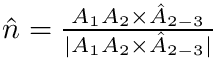where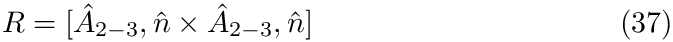is a torsional rotation force constant, , andis a torsion angle formed by the four bonded particles.

4. Secondary structure knowledge based torsion angle terms: in selected coarse grain models, where the covalent structure is a simple sequence of pseudo-atoms : SBL::CSB::T_Molecular_potential_energy_torsion_angle_in_linear_structure_term . For four successive particles, the formula is :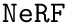where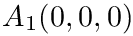are coefficients andis a torsion angle estimated from the four given particles.

## Bonded terms: the case of improper dihedral angles

Improper dihedral angles are defined in Molecular_coordinates. Such angles do not correspond to a physical interaction between the four atoms involved; instead, they are used to enforce planarity constraints e.g. in cycles.

The difficulty with such angles is the lack of a standard. To see why, recall that for a given central atom sharing bonds to three neighbors, there are three different improper angles–see Molecular_coordinates. To make the exploitation of improper angles canonical, we proceed as follows, the rationale being to enforce geometric planarity:

• The three angles are computed and are sorted by increasing value.
• The first one for which the spring constants are defined is retained to evaluate the penalty.
Due to the lack of a standard, we proceed as follows:

• Function SBL::CSB::T_Molecular_covalent_structure::add_improper_constraint allows the user to specify the improper angles for the system studied.
• Internally, only these specified angles are processed with the harmonic penalty.

## Non bonded terms

There are two classes provided for computing the different non bonded terms :

1. To compute the vdW term : SBL::CSB::T_Molecular_potential_energy_Lennard_Jones_term . For two non bonded particles, the formula is :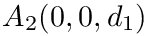where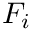is a constant,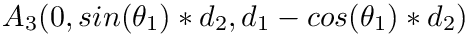are the repulsive and attractive Lennard-Jones terms, andis the distance between particles.

The functor has a distance threshold parameter to limit the number of unbonded pairs included in the calculation. This is achieved by means of a C++ visitor selecting pairs whose distance is less than the threshold.

To compute a simplified electrostatic term : SBL::CSB::T_Molecular_potential_energy_electrostatic_term . For two non bonded particles, the formula is :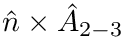whereis a constant,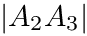are the charges of the two particles, andis the distance between particles.

## Molecules and solvent

Non protein molecules as well as atoms (ions) can be added into the covalent structure defined in the package Molecular_covalent_structure. This can be done in two ways:

• at the application level, by listing the molecules in the file describing the molecular system of interest.

• at the coding level, by directly adding the molecules of interest into the data structure representing the system of interest – see the package Molecular_covalent_structure for manually adding particles to a covalent structure.

# Format to represent force fields

We use the FFXML format to represent force fields.

In order to generate the FFXML files, it is necessary to convert the various existing formats with different scripts. For example, Amber and CHARMM have different representations of the force fields. In addition, depending on the molecule to study (sugar, peptide, protein, ...), and in which conditions they are presented (e.g, with or without solvant) , the parameters to load will be different.

To do so, we first resort on existing scripts if any, or define our own scripts otherwise.

## ffxml Format

The FFXML format (for Force Field XML format) is a simplified and hierarchical format for representing a given force field. It can be manually written for simple force fields as in section BLN69 , or it can be automatically created using the OpenMM library or the SBL scripts. For each case, the procedure for creating such an XML file is described in its own section.

The global architecture of a FFXML file is as follows :

<ForceField>
<AtomTypes>
</AtomTypes>
<Residues>
</Residues>
<HarmonicBondForce>
</HarmonicBondForce>
<HarmonicAngleForce>
</HarmonicAngleForce>
<PeriodicTorsionForce>
</PeriodicTorsionForce>
<NonbondedForce coulomb14scale="1.0" lj14scale="1.0">
</NonbondedForce>
</ForceField>


Depending on the input force field, some tags may appear or disappear. For example, an Amber force field is represented here in the FFXML format : Amber in FFXML.

Number types in force field specifications. Numerical values used in force fields are typically floating point numbers, but may also be rational numbers. For example, in the BLN69 Force Field, one finds a value of 2/3 for Lennard-Jones terms. This calls for three comments :
• reading a rational value is possible since our loader uses CGAL rational number type;

• the previous rational value is converted to a floating-point representation since calculations whose result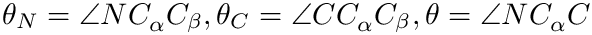are used in energy calculations;

• despite this conversion, using rational values avoids using an arbitrary number of digits in the file, so that the final precision is solely machine / number type dependent.

## Generating parameter files for force fields: Amber

The OpenMM library provides a Python script processAmberForceField.py for generating FFXML files from Amber files. Generating a FFXML file for amino acids is simply done with the following command :

processAmberForceField.py amber16/dat/leap/lib/all_amino03.lib amber16/dat/leap/lib/all_aminoct03.lib amber16/dat/leap/lib/all_aminont03.lib amber16/dat/leap/parm/parm10.dat > amber16.ffxml


The first three files describe the topology of the different amino acids of a peptidic chain, in the middle, at the C-terminus and at the N-terminus. The last file describes all the bonded / non-bonded parameters.

## Generating parameter files for force fields: CHARMM

The SBL provides the Python script sbl-process-CHARMM-force-field.py based on the ParmEd library. As for Amber, it loads CHARMM parameter files and generates a FFXML file based on the input parameters :

sbl-process-CHARMM-force-field.py toppar/top_all36_prot.rtf toppar/par_all36_prot.prm


The first file describes the topology of the different amino acids of a peptidic chain (at any position). The last file describes all the bonded / non-bonded parameters.

## Generating parameter files for force fields: Martini

The Martini force field is provided in the Gromacs format. The SBL provides the Python script sbl-process-Martini-force-field.py for processing Martini force fields in such a format. It takes as input a itp file defining the topology and the bonded / non-bonded parameters :

sbl-process-Martini-force-field.py martini_v2.2P_aminoacids.itp


## Generating parameter files for force fields: BLN69

The number of parameters being very small, and uniquely identified, we do not provide any script for generating the corresponding FFXML. We rather provide the corresponding FFXML file : BLN69 Force Field

# Examples: instantiating classical force fields

## Overview

The following examples computes the potential energy with different force fields, following the same pattern :

• Step 1: loading a list of conformations described in a text file listing PDB files;
• Step 3: creating the covalent structure of the input conformations based on an input PDB file;
• Step 4: computing the different contributions to the potential energy for each loaded conformation.

## Amber

Amber refers to a suite of force fields, see Amber. We consider here the ff14sb force field from Amber. The 6-tuples of this force filed are:

•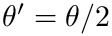parameters.
•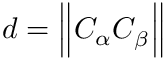unique parameters.

Note that there is no Lennard-Jones contribution in this force field. Note also that the charge is defined separately for each particle type, explaining the high number of parameters for the electrostatic contribution.

The ffxml file of the ff14sb force field is given here : ff14sb Amber Force Field.

The following example instantiates the Amber potential energy using the included file Force_field_atomic.hpp (see section Advanced : defining potential energy types for the specifications) :

## CHARMM

CHARMM refers to a suite of force fields, see CHARMM. We consider here the charmm36 force field for proteins. The 6-tuples of this force filed are

•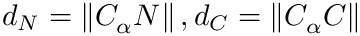parameters.
•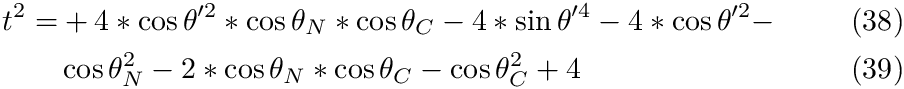unique parameters.

The ffxml file of the charmm36 force field is given here : CHARMM Force Field.

Note that the example of section Amber works also for this type of force field since the data structures are exactly the same.

## Martini

The Martini force field is a coarse grain force field designed to be used with Gromacs . The 6-tuples of this force filed are

•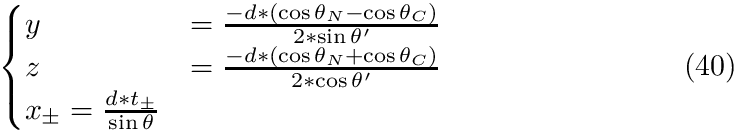parameters.
•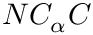unique parameters.

The ffxml file of the Martini force field is given here : Martini Force Field. In order to use the Martini force field in the SBL, two steps are required:

• converting the Gromacs itp files of the Martini force fields (this is done using the SBL script sbl-process-Martini-force-field.py)
• generating the coarse grain models to be used with these force fields .

The following example instantiates the Martini potential energy :

## BLN69

BLN69 is a protein model based on three types of particles, each representing a pseudo amino-acid – see Example Landscapes defined from mathematical functions . There is a specific force field based on the coarse grain model of BLN69. The 6-tuples of this force filed are

•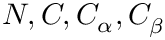parameters.
•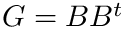unique parameters.

Note that there is a unique set of parameters for bonds and for angles. Note also that neither dihedral nor electrostatic terms are present in this force field.

See the BLN69 Force Field. See Example Landscapes defined from mathematical functions for more details about BLN69.

The following example instantiates the BLN69 potential energy :

# Advanced : defining potential energy types

This section introduces the different types defining the potential energy, used in the examples above.

## Simple potential energy definition

In the sequel, we take for granted the enumeration of primitive internal coordinates, as explained in the package Molecular_coordinates.

Three important types of C++ data structure are defined in this package. Following definition fig-eq-ff, contributions are defined from terms which are themselves defined from visitors:

Visitor : a visitor is a class defining an iterator over a collection of entities, entities being pairs, triples or quadruples of particles. An entity can represent an non-bonded pair of particles, a bond, a valence angle or a torsion angle. Visitor provided by the SBL are templated by the type CovalentStructure representing the type of the covalent structure (see package Molecular_covalent_structure). It defines the type Iterator representing the iterator itself, and the static methods begin( CovalentStructure ) and end( CovalentStructure ) for starting/ending the iteration over the target entities.

Term : a term is a functor computing one term of the sum of a contribution offor a specific entity (pair, triple or quadruple of particles). Terms provided by the SBL are templated by the type CovalentStructure representing the type of the covalent structure, the type ConformationType representing the geometric model (see package ConformationTraits), and the type PotentialEnergyParameters defining all the force fields parameters that are used by the term.

• Example : computing the term of one bond length is done using the functor SBL::CSB::T_Molecular_potential_energy_bond_length_term_harmonic< ConformationType , CovalentStructure , PotentialEnergyParameters >. This functor takes as input a conformation, a covalent structure and a bond representation in the covalent structure CovalentStructure::Bond_rep.
• Example : computing the term of one bond angle is done using the functor SBL::CSB::T_Molecular_potential_energy_bond_angle_term_harmonic< ConformationType , CovalentStructure , PotentialEnergyParameters >. This functor takes as input a conformation, a covalent structure and a bond angle representation in the covalent structure CovalentStructure::Bond_angle_rep.

Contribution : a contribution is a functor computing the contribution ofrelated to a term, over all the entities used by this contribution. There is a base generic contribution class SBL::CSB::T_Molecular_potential_energy_contribution< Visitor , Term > for instantiating any contribution from a unique term and a unique visitor.

Instantiating a potential energy function consists then in defining different contributions, and to sum them. For example, the class SBL::CSB::T_Molecular_potential_energy_bonded_contribution instantiates the three contributions related to the bond distances, bond angles and torsion angles, and sum them up. It is possible to instantiate in addition the Lennard Jones contribution from the classes SBL::CSB::T_Molecular_potential_energy_Lennard_Jones_term and SBL::CSB::T_Unbonded_particles_visitor for computing a basic potential energy without electrostatic term. Adding the electrostatic contribution is done in the same way by using the class SBL::CSB::T_Molecular_potential_energy_electrostatic_term

Computing the contribution of all bond lengths is done by defining the visitor SBL::CSB::T_Bonded_particles_visitor< CovalentStructure > and the term SBL::CSB::T_Molecular_potential_energy_bond_length_term_harmonic< ConformationType , CovalentStructure , PotentialEnergyParameters >. Then, the functor SBL::CSB::T_Molecular_potential_energy_contribution< Visitor , Term > with the appropriate types defines the contribution of all bonded particles.

typedef SBL::CSB::T_Bonded_particles_visitor<Covalent_structure> Visitor;
typedef SBL::CSB::T_Molecular_potential_energy_bond_length_term<Conformation,
Covalent_structure,
Parameters> Term;
typedef SBL::CSB::T_Molecular_potential_energy_term<Visitor, Term> Term;
Conformation C;
Covalent_structure S;
Contribution contrib;
double e = contrib(C, S);

Computing the Lennard-Jones contribution is done by defining the visitor SBL::CSB::T_Unbonded_particles_visitor< CovalentStructure > and the term SBL::CSB::T_Molecular_potential_energy_Lennard_Jones_term< ConformationType , CovalentStructure , PotentialEnergyParameters >. Then, the functor SBL::CSB::T_Molecular_potential_energy_contribution< Visitor , Term > with the appropriate types defines the Lennard-Jones contribution.

typedef SBL::CSB::T_Unbonded_particles_visitor<Covalent_structure> Visitor;
typedef SBL::CSB::T_Molecular_potential_energy_Lennard_Jones_term<Conformation,
Covalent_structure,
Parameters> Term;
typedef SBL::CSB::T_Molecular_potential_energy_contribution<Visitor, Expression> Contribution;
Conformation C;
Covalent_structure S;
Contribution contrib;
double e = contrib(C, S);


## Conformation related types definitions

We need to define the types to represent a conformation, and to load conformations from an input file.

#include <SBL/Models/Geometric_conformation_traits.hpp>
typedef SBL::Models::T_Geometric_conformation_traits<>         Conformation_traits;
typedef Conformation_traits::Conformation                      Conformation;


## Covalent structure related types definitions

Four types have to be defined :

• the representation of a particle in the covalent structure : it will be used for identifying a particle in the covalent structure so that a force field parameter can be easily associated to a given particle;
• the covalent structure type : it is the main data structure, that is a wrapper for a graph representing the topology of the protein;
• the covalent structure builder : it fills a covalent structure from an arbitrary data structure built from a loaded file;
• the covalent structure loader : it loads the covalent structure from a PDB file into an arbitrary data structure, then uses the builder for filling the desired covalent structure object.
#include <SBL/CSB/Particle_info_for_proteins.hpp>
#include <SBL/CSB/Molecular_covalent_structure.hpp>
typedef SBL::CSB::T_Particle_info_for_proteins<>                                                                     Particle_info;
typedef SBL::CSB::T_Molecular_covalent_structure<Particle_info>                                                      Covalent_structure_base;
typedef SBL::CSB::T_Molecular_covalent_structure_builder_for_proteins<Covalent_structure_base>                       Covalent_structure_builder;


## Potential energy parameters

All the parameters used to compute the potential energy function are gathered in the class SBL::IO::T_Potential_energy_parameters< CovalentStructure , UnitSystemTraits > : these parameters corespond to the force field parameters, and to the units used to represent the constants in the force field parameters. The parameter CovalentStructure is a covalent structure as defined in the package Molecular_covalent_structure, and the parameter UnitSystemTraits defines the unit system to be used, as defined in the package UnitSystemTraits.

This class also provides a loader for the ffxml files, and gives a naive acces to the corresponding parameters. Instantiating this class can be done simply like this :

#include <SBL/IO/Potential_energy_parameters.hpp>
#include <SBL/Models/Unit_system_traits_none_AKMA.hpp>
#include <SBL/Models/Unit_system_traits_for_potential_energy.hpp>
#include <SBL/CSB/Molecular_potential_energy_structure_parameters_traits_default.hpp>

typedef SBL::Models::T_Unit_system_traits_none_AKMA<double>                        UST_base;
typedef SBL::Models::T_Unit_system_traits_for_potential_energy<UST_base>           UST;


## Optimized data structures

The way parameters are built and the access to the covalent structure data structure can be time consuming, in particular in the context of computing potential energy and its gradient. To circumvent this difficulty, it is possible to use optimized data structures, with less functionality but more efficient access :

• SBL::CSB::T_Potential_energy_parameters_optimal, representing the optimized version of the parameters class.

Once the base covalent structure and the base parameters have been loaded, it is possible to build a new covalent structure object and a new parameters object with constant time access to each parameter, and with the possibility to visit only entities (particles bonds or angles) having a non-zero contribution to the potential energy.

In order to do so, the class SBL::CSB::T_Molecular_potential_energy_structure_parameters_traits_optimal< CovalentStructureBase , PotentialEnergyParametersBase > provides three important types :

• Covalent_structure, that represents the optimized version of the covalent structure class
• Potential_energy_parameters, that represents the optimized version of the parameters class
• Builder_structure_parameters, that is a functor taking the base covalent structure and the base parameters class, and returns the pair of corresponding optimized data structures.

In this way, the following code allows to simply define the optimal versions :

#include <SBL/CSB/Molecular_potential_energy_structure_parameters_traits_optimal.hpp>
typedef SBL::CSB::T_Molecular_potential_energy_structure_parameters_traits_optimal
typedef Structure_parameters_traits::Covalent_structure                            Covalent_structure;
typedef Structure_parameters_traits::Potential_energy_parameters                   Potential_energy_parameters;
typedef Structure_parameters_traits::Build_structure_parameters                    Build_structure_parameters;


In order to minimalize the code changes to switch from one representation to the other, the class SBL::CSB::T_Molecular_potential_energy_structure_parameters_traits_default is also provided :

• the type Covalent_structure is the base covalent structure class
• the type Potential_energy_parameters is the base parameters class
• the type Builder_structure_parameters simply returns the two input objects.
#include <SBL/CSB/Molecular_potential_energy_structure_parameters_traits_default.hpp>
typedef SBL::CSB::T_Molecular_potential_energy_structure_parameters_traits_default
typedef Structure_parameters_traits::Covalent_structure                            Covalent_structure;
typedef Structure_parameters_traits::Potential_energy_parameters                   Potential_energy_parameters;
typedef Structure_parameters_traits::Build_structure_parameters                    Build_structure_parameters;


## Potential energy : composed terms;

Two important types have to be defined :

• the bonded contribution of the potential energy : computes the sum of all bonded contributions, corresponding to the first four entries in the 6-tuple above. Note that it is possible to compute separately each contribution, at the cost of some more type definitions.
• the non bonded contributions of the potential energy : compute the vdw and electrostatic contributions, corresponding to the 5th and 6th terms in the 6-tuple above.
#include <SBL/CSB/Molecular_potential_energy.hpp>

typedef SBL::CSB::T_Molecular_potential_energy_bonded_contribution
<Conformation, Covalent_structure, Potential_energy_parameters>                                        Bonded_contributions;

typedef SBL::CSB::T_Unbonded_particles_visitor<Covalent_structure>                                     Non_bonded_visitor;
typedef SBL::CSB::T_Molecular_potential_energy_Lennard_Jones_term
<Conformation, Covalent_structure, Potential_energy_parameters>                                        Lennard_Jones_term;
typedef SBL::CSB::T_Molecular_potential_energy_electrostatic_term
<Conformation, Covalent_structure, Potential_energy_parameters>                                        Electrostatic_term;

typedef SBL::CSB::T_Molecular_potential_energy_contribution
<Non_bonded_visitor, Lennard_Jones_term, Electrostatic_term>                                           Non_bonded_contributions;


## Dumping specific potential energy terms into a file

We have seen above that adding up terms of a given types makes up a contribution. Sometimes, it is useful to inspect closely all terms of a given type. As an example, energy type refers to the Lennard Jones potential in the sequel.

We now describe the mechanism implemented to dump all such terms into a file, in four steps.

First, the class SBL::CSB::T_Molecular_potential_energy_term_static_info defines a static variable indicating that all terms of a given energy type must be dumped into a file, and the corresponding filestream. Note that the class is templated by a TermType: we indeed want the aforementioned variables to exist for this specific energy type only.

Second, the term of interest, SBL::CSB::T_Molecular_potential_energy_Lennard_Jones_term, in our case, inherits from this static class. To instantiate the class SBL::CSB::T_Molecular_potential_energy_term_static_info , we use the Curiously recurring template pattern.

Third, the functor of the energy type, SBL::CSB::T_Molecular_potential_energy_Lennard_Jones_term , does the energy computation and if the flag is set, dumps the result into a file.

Fourth, the flags and the output file name are set in the main application, as static members:

  Lennard_Jones_term::s_dump_flag = true;
Lennard_Jones_term::s_ofname = "/tmp/LJ-terms.txt";
Lennard_Jones_term::s_ostream;


Naturally, the values of these static variables can be set using boost::program_options. As an example, checkout sbl-energy-charmm.{cpp,exe}. The following call generate the file /tmp/LJ-terms.txt containing LJ terms for all non bonded pairs:

sbl-energy-charmm.exe -c ala5.txt -s ala5.pdb -p charm.xml --energy-terms


For each potential energy term, this package provides also an associated gradient term. Recall that potential energy terms are expressed with internal coordinates, while the input is expressed with cartesian coordinates. Therefore a potential energy term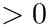is the composition of two functions, namely the conversion from cartesian to internal coordinates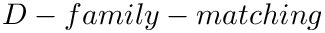, and the actual potential energy calculation from internal coordinates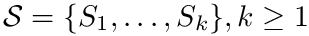. Therefore, the multivariate chain rule yields: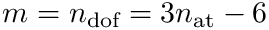This package provides gradients for the functions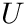and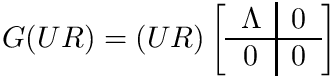. The different formulae of the gradients are computed using the Python package for symbolic mathematics sympy, and then injected into the C++ code.